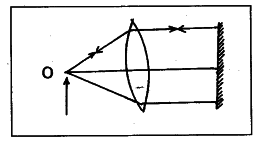# The ray diagram given below illustrates

The ray diagram given below illustrates the experimental set up for the determination of the focal length of a converging lens using a plane mirror.

1. State the magnification of the image formed.
2. Write the characteristics of the image formed.
3. What is the name given to the distance between the object and optical centre of the lens in the following diagram ?1. The magnification of the image formed is unity (or 1).
2. The image is (a) real and (b) inverted is at the same position of the object.
3. The distance between the object and the optical centre of the lens is called object distance.